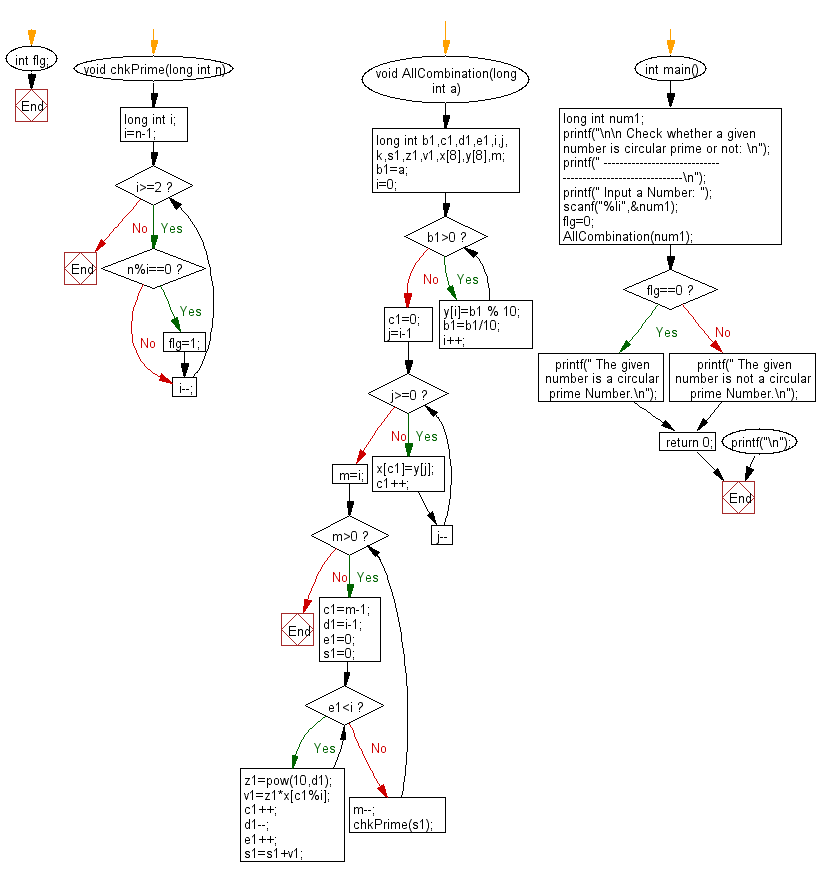﻿ C Program: Check if a given number is circular prime or not - w3resource# C Exercises: Check if a given number is circular prime or not

## C Numbers: Exercise-27 with Solution

Write a program in C to check if a given number is circular prime or not.

Test Data
Input a Number: 1193

Sample Solution:

C Code:

``````# include <stdio.h>
# include <stdlib.h>
# include <stdbool.h>
# include <math.h>

int flg;
void chkPrime(long int n)
{
long int i;
i=n-1;
while(i>=2)
{
if(n%i==0)
{
flg=1;
}
i--;
}
}
void AllCombination(long int a)
{
long int b1,c1,d1,e1,i,j,k,s1,z1,v1,x,y,m;
b1=a;
i=0;
while(b1>0)
{
y[i]=b1 % 10;
b1=b1/10;
i++;
}
c1=0;
for(j=i-1;j>=0;j--)
{
x[c1]=y[j];
c1++;
}
m=i;
while(m>0)
{
c1=m-1;
d1=i-1;
e1=0;
s1=0;
while(e1<i)
{
z1=pow(10,d1);
v1=z1*x[c1%i];
c1++;
d1--;
e1++;
s1=s1+v1;
}
m--;
chkPrime(s1);
}
}
int main()
{
long int num1;
printf("\n\n Check whether a given number is circular prime or not: \n");
printf(" -----------------------------------------------------------\n");
printf(" Input a Number: ");
scanf("%li",&num1);
flg=0;
AllCombination(num1);
if(flg==0)
{
printf(" The given number is a circular prime Number.\n");
}
else
{
printf(" The given number is not a circular prime Number.\n");
}
return 0;
printf("\n");
}
```
```

Sample Output:

``` Input a Number: 1193
The given number is a circular prime Number.
```

Pictorial Presentation:Flowchart:C Programming Code Editor:

What is the difficulty level of this exercise?

Test your Programming skills with w3resource's quiz.

﻿

## C Programming: Tips of the Day

Why do C and C++ compilers allow array lengths in function signatures when they're never enforced?

It is a quirk of the syntax for passing arrays to functions.

Actually it is not possible to pass an array in C. If you write syntax that looks like it should pass the array, what actually happens is that a pointer to the first element of the array is passed instead.

Since the pointer does not include any length information, the contents of your [] in the function formal parameter list are actually ignored.

Ref : https://bit.ly/3fhlvdH